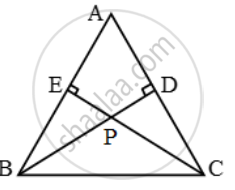# In figure, considering triangles BEP and CPD, prove that BP × PD = EP × PC. - Mathematics

Sum

In figure, considering triangles BEP and CPD, prove that BP × PD = EP × PC.

#### Solution

Given: A ∆ABC in which BD ⊥ AC and CE ⊥ AB and BD and CE intersect at P.

To Prove: BP × PD = EP × PC

Proof: In ∆EPB and ∆DPC, we have

∠PEB = ∠PDC [Each equal to 90º]

∠EPB = ∠DPC [Vertically opposite angles]Thus, by AA-criterion of similarity, we have

∆EPB ~ ∆DPC

\frac{EP}{DP}=\frac{PB}{PC}

⇒ BP × PD = EP × PC

Concept: Similarity
Is there an error in this question or solution?